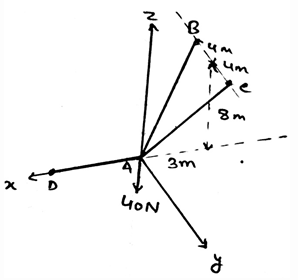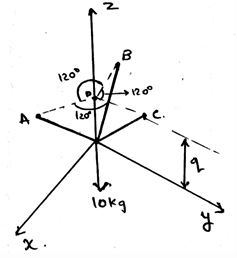Courses

# Test: Potential Energy Criterion For Equilibrium

## 15 Questions MCQ Test Engineering Mechanics | Test: Potential Energy Criterion For Equilibrium

Description
This mock test of Test: Potential Energy Criterion For Equilibrium for Mechanical Engineering helps you for every Mechanical Engineering entrance exam. This contains 15 Multiple Choice Questions for Mechanical Engineering Test: Potential Energy Criterion For Equilibrium (mcq) to study with solutions a complete question bank. The solved questions answers in this Test: Potential Energy Criterion For Equilibrium quiz give you a good mix of easy questions and tough questions. Mechanical Engineering students definitely take this Test: Potential Energy Criterion For Equilibrium exercise for a better result in the exam. You can find other Test: Potential Energy Criterion For Equilibrium extra questions, long questions & short questions for Mechanical Engineering on EduRev as well by searching above.
QUESTION: 1

### If solving the question in 3D calculations is difficult, then use the 2D system and then equate the total net force to zero as the criteria of the potential energy for equilibrium.

Solution:

The answer is obviously yes. If we are having any difficulty in making the vector components, then we can go in 2D. As if the particle is in equilibrium, the net force will be zero. No matter where you see first. Net force is zero.

QUESTION: 2

### If the body is upward the ground then the distance taken is __________

Solution:

The direction upwards is considered as positive. And then the calculations are done. Not only that the direction of the rotation is also concerned with the signs. Positive if on the positive z axis. Negative if towards negative z axis.

QUESTION: 3

### If the resolved force or the force which you get as the answer after solving the question is negative for the equilibrium of the potential energy, then what does this implies?

Solution:

The negative sign implies things in the opposite manner. If the force is coming negative this doesn’t means that it is impossible. But it means that the force is in the opposite direction w.r.t the direction set by you in the free body diagram.

QUESTION: 4

A force is developed by a support that not allows the ________ of its attached member in the calculation of the potential energy.

Solution:

The force developed by a support doesn’t allow the translation of its attached member. This is the basic condition for the equilibrium of the forces in any dimension. This rule is applied when the support reactions are taken into the account for the equilibrium of the body.

QUESTION: 5

We first make equilibrium equations of the body by considering all the three dimensional forces and then the free body diagram is made and solved. Thus the criteria for the potential energy equilibrium.

Solution:

We first make the free body diagram and then we make the equilibrium equations to satisfy the given conditions. This helps us to solve the question easily. As this reduces the part of imagination and increases accuracy too.

QUESTION: 6

Couple moment is also considered as one of the criteria for the equilibrium of the potential of the body. It should be zero sometimes. So a couple moment is developed when _______ of the attached member is prevented.

Solution:

The development of the couple moment is when there is prevention of the rotation of the attached member. This is the basic condition for the equilibrium of the couple moments in any dimension. This rule is applied when the couple moments are taken into the account for the equilibrium of the body.

QUESTION: 7

Find the tension in the cable AC.Solution:

First represent the forces in their vector form. Then equate the net sum of the forces in the x, y and z directions to be zero. You will get FB = FC and 2(.848) = 40N. This gives the answer as 23.6N.

QUESTION: 8

If the supports are properly aligned then the reaction forces developed under the potential energy diagrams are adequate to support the body.

Solution:

This is the basic nature observed during the experiments on the beams an all the support system. The dimension doesn’t affect the equilibrium conditions. The main motto is to achieve the equilibrium. It is achieved by equating the net force equal to zero.

QUESTION: 9

What is not the criteria for the equilibrium in potential energy free body diagram?

Solution:

For the equilibrium in the three dimensional system of axis we have all the conditions true as, ∑Fx=0, ∑Fy=0 and ∑Fz=0. Also we have the summation of the forces equal to zero. Which is not a non-zero value.

QUESTION: 10

If any body is tied to three or more ropes and is in the potential equilibrium, and then is allowed to achieve its equilibrium. Then the equilibrium achieved is achieved w.r.t what?

Solution:

Yes, the equilibrium is being achieved w.r.t the ground. Like the motion w.r.t ground need be zero. That is the relative velocity of the object or the body must be zero w.r.t the ground. This means motion is in equilibrium.

QUESTION: 11

∑Fx=0, ∑Fy=0 and ∑Fz=0 are vector equations for the three dimensions. They are satisfied when the body is achieved it state of equilibrium and the body has potential energy stabilised.

Solution:

The answer is false as the equations asked are scalars. As we make the net sum of the forces along the axis equal to zero. Of course this equation comes from the solving the vector forms, but still the result is a scalar, hence the equations are scalar.

QUESTION: 12

The supports in the 3D are having more than three reaction forces. Because they are having three axis on which the components of the forces need to be zero, so as to equilibrate the potential energy.

Solution:

As 3D is defined as the three axis system, we have to consider the equilibrium in all the three axis. This will make the equilibrium go on all the axis of the 3D space. And hence will cancel all the forces.

QUESTION: 13

The single pin, single bearing and single hinge resist _______ so as to attain potential energy equilibrium.

Solution:

The experiments have founded that the single pin, single bearing and single hinge resist both force and couple moments. This does helps in attaining the equilibrium of the body. Thus proper guiding must be taken during solving the question on the same.

QUESTION: 14

Determine the value of the q, parallel to the z axis. That is the point of intersection of the projections of the points A, B and C parallel to the xy plane. With the distance between the tri-section point and the points A, B and C be equal to 0.6m.Solution:

The application of the equilibrium equation will yield the result. That is the resultant along the z-axis will remain zero. Which give the value of γ as 50˚. And therefore q=51.9cm.

QUESTION: 15

If the supports which are supporting a body at a certain height are not properly aligned then the reaction forces developed are adequate to support the body.

Solution:

This is the basic nature observed during the experiments on the beams an all the support system. The dimension doesn’t affect the equilibrium conditions. The main motto is to achieve the equilibrium. It is achieved by equating the net force equal to zero. But here the system is not properly aligned.